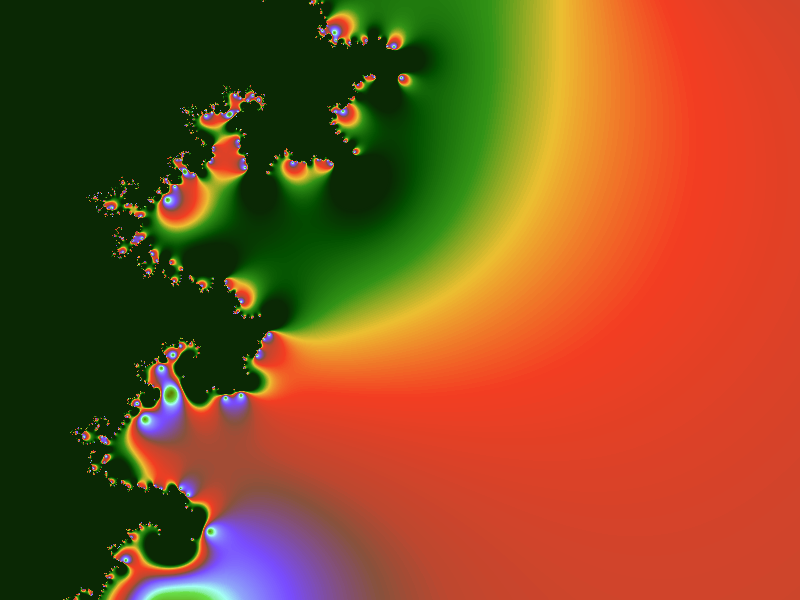# pheelicks

This post is part of a series. For a listing of all the posts, as well as instructions on running the code, see here.

The last couple of posts have been more about laying some foundations on how to graphically display output from our Go program in real-time, and have focused primarily on the networking side of things. So I thought it was probably only fair to play around some more with pretty pictures. Here’s one: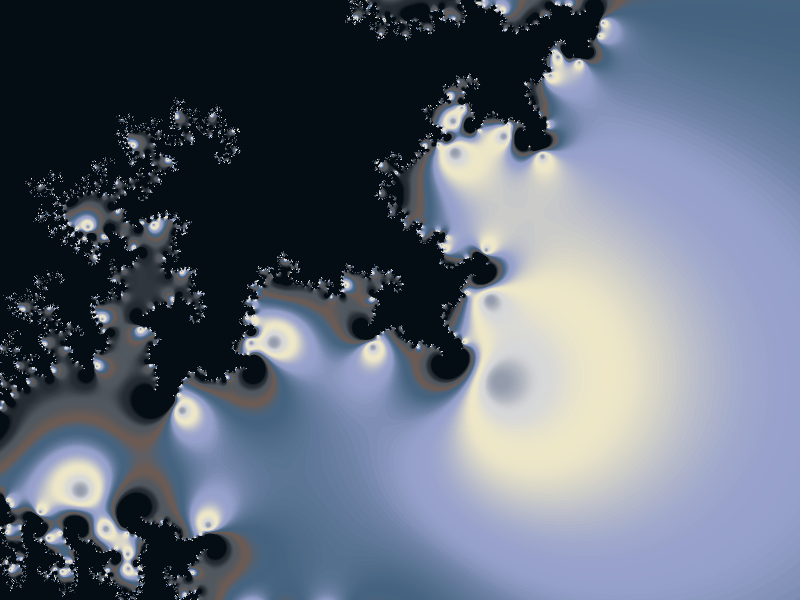## Fractals

If you’re mathematically inclined, you should recognize this as a fractal. If you’re mathematically obsessed, you will recognize this as the Mandelbrot set at `-0.71 + -0.25i`.

For a full description of what the Mandelbrot set fractal is, have a read on Wikipedia, but all you really need to know for the purposes of this post is that is visualization of collection of complex numbers. Specifically, for each number `c` in the field we want to know if the following expression remains bounded when we iterate it:

``````z = z * z + c
``````

Depending on how quickly this expression diverges (or doesn’t) we will set the color of a pixel corresponding to `c`. The code for calculating `c` will look something like this:

``````z := complex(0, 0)
for i := 0; i < iter; i++ {
z = z*z + c
}
return cmplx.Abs(z)
``````

Actually, it will look exactly like that, as Go helpfully comes with complex numbers included.

Don’t worry if you don’t understand complex numbers, the above code is pretty much the only place they are used. Every time a complex number `c` is passed in that code will spit out a magnitude which corresponds to a color on our image, e.g. a low value might be black, while a high value white.

OK, so now we know how we’ll calculate the value for a single complex number, how do we get from there to a pretty picture? Like this one: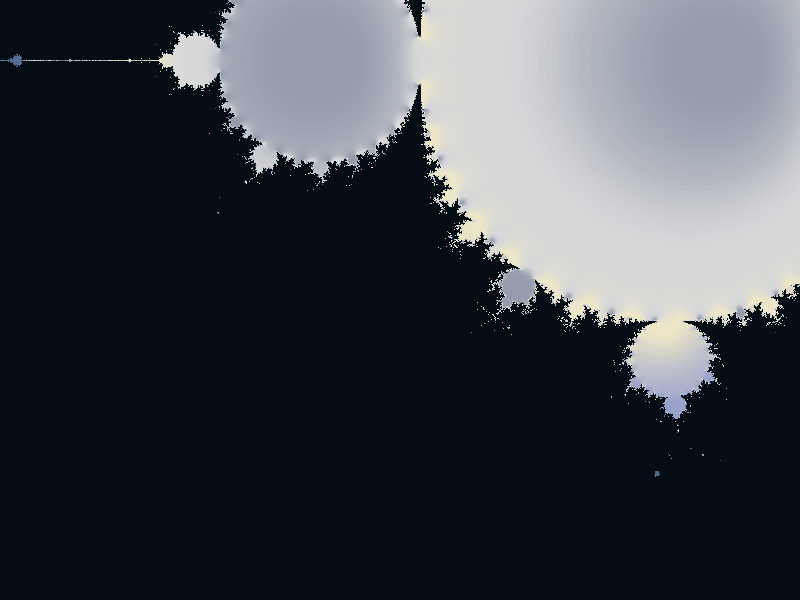## Coordinate systems

At a high level, we’ll want to create a Canvas, and then iterate over every pixel in that canvas, calculating the value to which our Mandelbrot expression diverges to. However, there are two other parameters that we need to pass in. To be able to pan around the fractal and zoom into it, we need to also specify the `zoom` and the `center`.

The `zoom` parameter corresponds to how many pixels we should draw per unit in the complex plane. So a factor of 10 means that in the range `0 -> 1` we will have 10 pixels.

The `center` parameter is the offset of our Canvas. In other words what value of `c` should the pixel in the middle of our Canvas correspond to.

Converting between a coordinate `x, y` in our Canvas at zoom level `zoom`, centered at `center` is fairly straightforward, we just scale linearly by `zoom` and then add on the `center` coordinate:

``````func toCmplx(x, y int, zoom float64, center complex128) complex128 {
return center + complex(float64(x)/zoom, float64(y)/zoom)
}
``````

``````zoom := 100.0
center := complex(-0.21, 0.25)
size := canvas.Bounds().Size()
for x := 0; x < size.X; x++ {
for y := 0; y < size.Y; y++ {
c := toCmplx(x-size.X/2, y-size.Y/2, zoom, center)
mag := mandelbrot(c, 50)
color := colorizer(mag)
canvas.Set(x, y, color)
}
}
``````

Simple. The only subtlety is that when we pass in out `x` and `y` values, we need to measure them relative to the center of the Canvas, which is why you’ll see `x-size.X/2` and `y-size.Y/2`.

However, one piece is still missing: how do we go from a magnitude value to a color?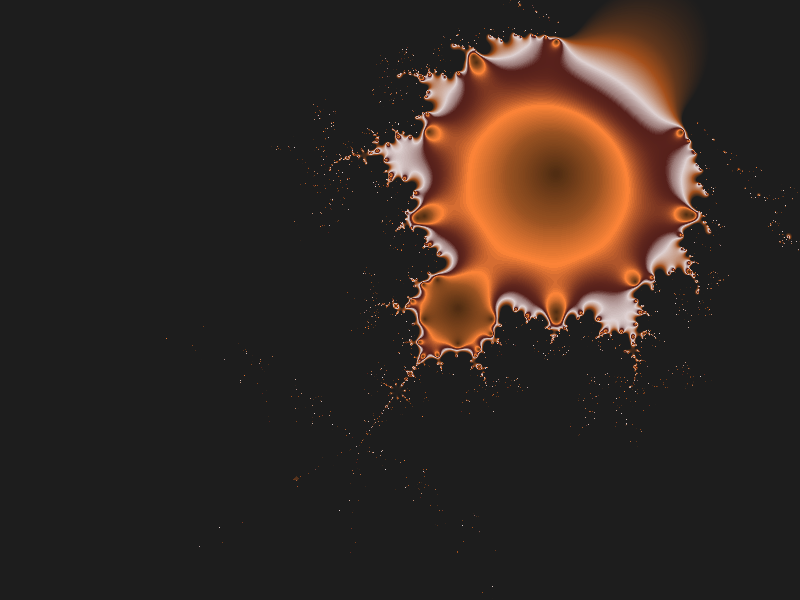## Colorizer

The simplest way to go from a value to a color is to just map the value directly to a color channel, e.g. 0 is black and 100 is red. However this makes for boring looking images. Much better is to create a gradient image and use that to assign the colors. These gradients can easily be created in most image editing programs, or you can use one of these.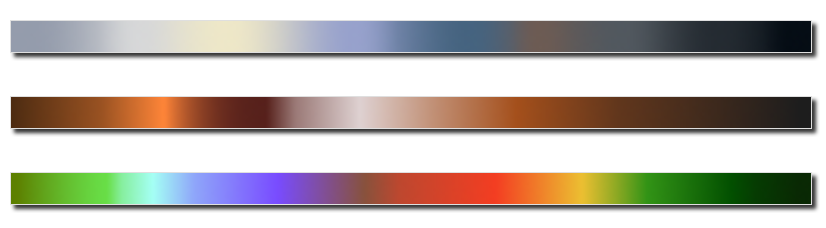We already know how to load in images from earlier posts, but to streamline it I’ve created a utility method to create a Canvas directly from a filename. Check out canvas.go for details.

Now onto making that colorizer. As the function is pretty simple, rather than creating a whole new type, we’ll just use the closure support in Go to create a function using another function. Specifically, we’ll have a `createColorizer` function which we’ll pass a filename for our gradient, and it will return a function, `colorizer(mag float64)` which we’ll actually use for generating the correct colors.

``````func createColorizer(filename string) func(float64) color.Color {
return func(mag float64) color.Color {
// Clamp magnitude to size of gradient
m := int(math.Max(math.Min(300*mag, float64(limit)), 1))
}
}
``````

Really all the colorizer does is that it takes the value of the magnitude `mag` and looks up the color of the pixel corresponding to that value in the gradient image. There is also a bit of code that ensures that we don’t try and look up pixels outside the bounds of the gradient.

One thing to note is that we are accessing `gradient` inside the colorizer function, even though it is defined in `createColorizer`. The value of `gradient` has been bound in the closure of the returned `func`, so we can use it every time we want to color a pixel, however we will not have to read in the gradient image file again and again.

This is different from if we had defined `gradient` as a global variable. Because it is in the closure, we can create multiple colorizers with different gradients and they will each hold onto their own instance of the `gradient` variable. E.g. the following will produce two independent colorizers:

``````c1 := createColorizer("gradient1.png")
``````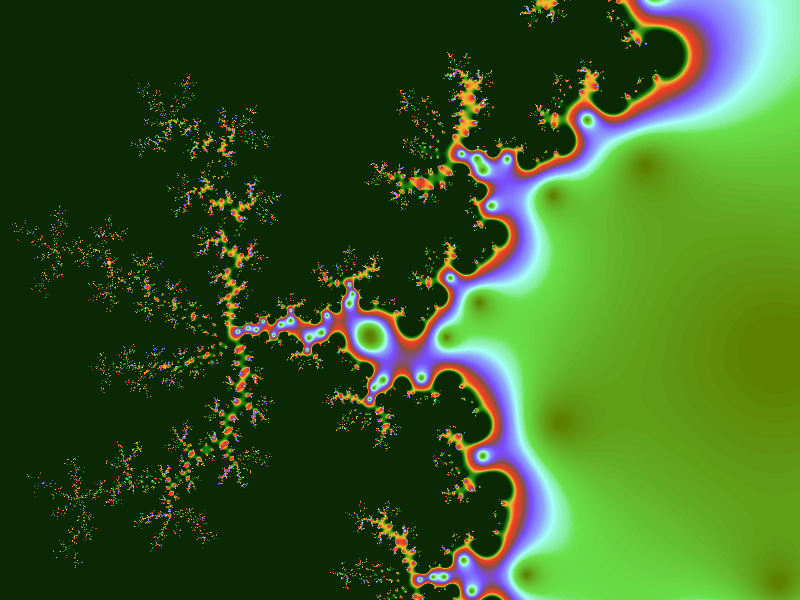## Playing around

To create some fractals of your own, check out the code on github.

To run the code, use `go run fractal.go canvas.go vector.go`

All the logic has been bundled up into a `drawFractal` function, which you can pass a `zoom`, `center` and a `colorizer`, and experiment with what comes out. I find it’s best to start zoomed out and center the image on what looks to an interesting location. Then increase the `zoom` and refine the `center`, until you get to an image you like.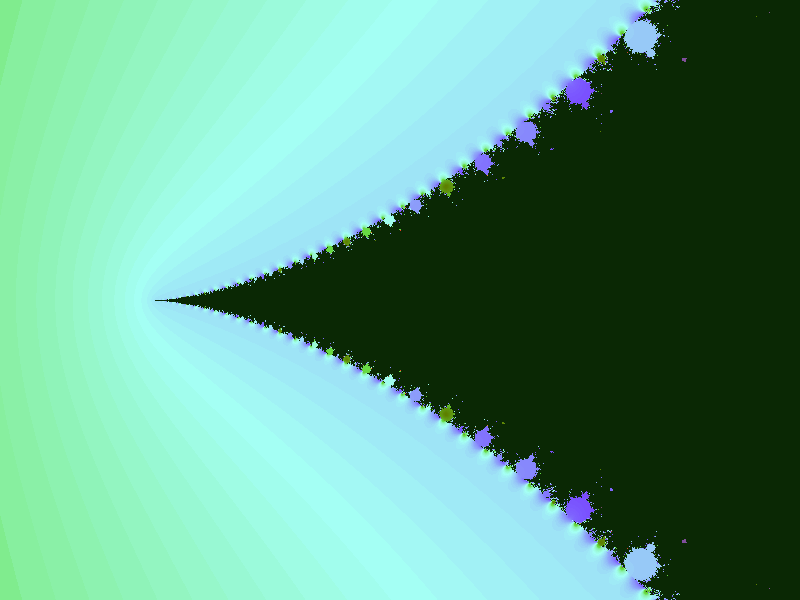Once you’ve found an interesting region, try recoloring with different gradients. You can also increase/decrease the number of iterations the `mandelbrot` function is to do before returning, which will control the level of detail present in the image.